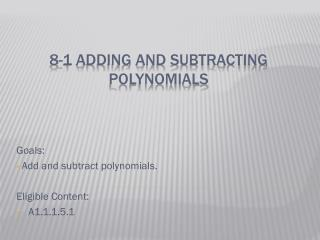DownloadDownload Presentation# 8-1 Adding and subtracting Polynomials

Télécharger la présentation## 8-1 Adding and subtracting Polynomials

- - - - - - - - - - - - - - - - - - - - - - - - - - - E N D - - - - - - - - - - - - - - - - - - - - - - - - - - -
##### Presentation Transcript

1. Goals: Add and subtract polynomials. Eligible Content: A1.1.1.5.1 8-1 Adding and subtracting Polynomials

2. Polynomial – a mathematical expression consisting of a sum of terms. Each term is the product of a constant and one or more variables raised to a positive or zero power. • Examples: Vocabulary

3. Standard Form – the terms are in descending order of exponents. vocabulary

4. Constant – degree is zero. Linear – degree is one. Quadratic – degree is two. Cubic – degree is three. Quartic – degree is four. vocabulary

5. Leading coefficient – the coefficient of the first term when the polynomial is in standard form. vocabulary

6. Monomial – polynomial with one term. Binomial – polynomial with two terms. Trinomial – polynomial with three terms. Anything bigger – polynomial vocabulary

7. Examples Give the degree and classify the polynomial by degree and number of terms. • -5 • ¼ x • -9x+2 • x2 – 6 • -x3 +2x + 1 • 3x4 +2x3 – x2 +5x – 8 • 0, constant, monomial • 1, linear, monomial • 1, linear, binomial • 2, quadratic, binomial • 3, cubic, trinomial • 4, quartic, polynomial

9. examples

10. Find (3x2 + 2x – 1) + (–5x2 + 3x + 4). A. –2x2 + 5x + 3 B. 8x2 + 6x – 4 C. 2x2 + 5x + 4 D. –15x2 + 6x – 4

11. Find (4x3 + 2x2 – x + 2) + (3x2 + 4x – 8). A. 5x2 + 3x – 6 B. 4x3 + 5x2 + 3x – 6 C. 7x3 + 5x2 + 3x – 6 D. 7x3 + 6x2 + 3x – 6